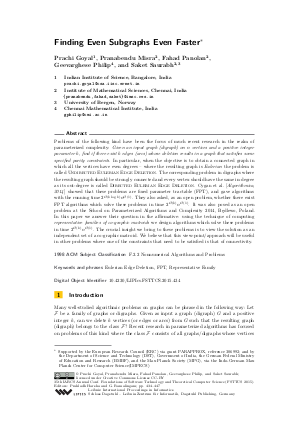Document# Finding Even Subgraphs Even Faster

### Authors Prachi Goyal, Pranabendu Misra, Fahad Panolan, Geevarghese Philip, Saket Saurabh## File

LIPIcs.FSTTCS.2015.434.pdf
• Filesize: 0.52 MB
• 14 pages

## Cite As

Prachi Goyal, Pranabendu Misra, Fahad Panolan, Geevarghese Philip, and Saket Saurabh. Finding Even Subgraphs Even Faster. In 35th IARCS Annual Conference on Foundations of Software Technology and Theoretical Computer Science (FSTTCS 2015). Leibniz International Proceedings in Informatics (LIPIcs), Volume 45, pp. 434-447, Schloss Dagstuhl - Leibniz-Zentrum für Informatik (2015)
https://doi.org/10.4230/LIPIcs.FSTTCS.2015.434

## Abstract

Problems of the following kind have been the focus of much recent research in the realm of parameterized complexity: Given an input graph (digraph) on n vertices and a positive integer parameter k, find if there exist k edges(arcs) whose deletion results in a graph that satisfies some specified parity constraints. In particular, when the objective is to obtain a connected graph in which all the vertices have even degrees--where the resulting graph is Eulerian,the problem is called Undirected Eulerian Edge Deletion. The corresponding problem in digraphs where the resulting graph should be strongly connected and every vertex should have the same in-degree as its out-degree is called Directed Eulerian Edge Deletion. Cygan et al.[Algorithmica, 2014] showed that these problems are fixed parameter tractable (FPT), and gave algorithms with the running time 2^O(k log k)n^O(1). They also asked, as an open problem, whether there exist FPT algorithms which solve these problems in time 2^O(k)n^O(1). It was also posed as an open problem at the School on Parameterized Algorithms and Complexity 2014, Bedlewo, Poland. In this paper we answer their question in the affirmative: using the technique of computing representative families of co-graphic matroids we design algorithms which solve these problems in time 2^O(k)n^O(1). The crucial insight we bring to these problems is to view the solution as an independent set of a co-graphic matroid. We believe that this view-point/approach will be useful in other problems where one of the constraints that need to be satisfied is that of connectivity.
##### Keywords
• Eulerian Edge Deletion
• FPT
• Representative Family.

## Metrics

• Access Statistics
• Total Accesses (updated on a weekly basis)
0